•5星
5.47MB zhangjin7422 2021-01-28 10:18:50
•5星
1.45MB zhangjin7422 2021-04-07 09:24:30
•5星
49KB weixin_58444518 2021-05-21 11:46:39
•3星
2.07MB sxxywmw 2019-01-16 12:42:18
• 10.28MB zzccool 2018-05-30 09:55:36
• VB程序设计教程:从入门到精通 教学 编程 设计

9KB weixin_26736139 2021-06-14 12:46:10
• 15KB m0_52957036 2020-04-16 06:27:09
• 7.96MB yyzleaves 2019-05-08 18:00:21
• 简单计算器vb程序设计 程序设计

68KB a_r_temis 2021-05-05 19:57:47
• 27KB m0_52957036 2020-12-01 00:07:30
• 317KB qq_29817063 2015-07-14 16:44:35
• 编程大众化-vb程序设计pdf文本版 编程语言

3.35MB qq_36916968 2020-08-22 15:06:59
• 60.93MB weixin_39649102 2019-05-15 16:08:42
• 3.03MB fisherxu 2017-03-07 17:37:02
• 有限单元法及程序设计(VB程序设计) 有限单元法及程序设计

32KB weixin_44554436 2020-11-18 15:50:21
• 16KB woshimawei123 2014-12-22 21:40:54
• 6.52MB m0_52957036 2020-04-09 08:07:52
•5星
7.12MB shenhaibing2000 2012-09-05 10:10:51
• 351KB m0_52957036 2020-10-23 07:46:03
• 20KB qq_41511934 2018-12-25 22:15:44
• 492KB Augusdi 2010-03-13 23:36:16
• 程序设计VB作业 VB程序设计作业 程序设计VB

648KB liguiong 2018-05-22 00:50:03
• 131B abcd229134522 2011-06-24 07:43:45
• vb程序设计案例教程 VB程序设计5星
18.83MB cll10213033 2014-05-13 19:44:03
• 637KB gouyue 2011-11-08 16:51:05
• VB程序设计练习题 vb6 编程语言 后端 经验分享

这是我备考技能考的一些VB练习题。（难度稍微有点大哦） 想要更多VB练习题点击我的头像，看我发表我文章就可以看到了。 答案是小编自己写的，也许会有错。 内容 一、明文密文转换。 在上面的文本框中输入的英文...

目录

文章目录

前言

内容

一、明文密文转换。

二、大小写互相转换。

三、三角形的综合问题。

五、标签左右移动。

六、相邻数加起来为素数。

七、通项分母(累加).

八、判断降序数。

九、产生数组，求最大数。

十、奇偶排序。

总结：

前言

这是我备考技能考的一些VB练习题。（难度稍微有点大哦）

想要更多VB练习题点击我的头像，看我发表我文章就可以看到了。

答案是小编自己写的，也许会有错。

内容

一、明文密文转换。

在上面的文本框中输入的英文字母（称为”明文")加密，加密结果（称为"密文")显示在下面的文本框中。加密的方法是：选中一个单选按钮，单击“加密”按钮后，根据选中的单选按钮后面的数字n，把明文中的每个字母改为它们后面第n个字母（"z"后面的字母认为是"a"，"Z"后面的字母认为是"A"），如图所示。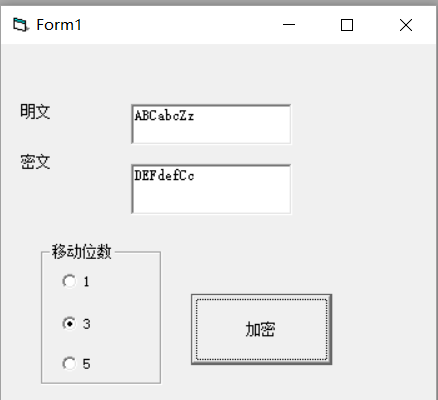答案：

Private Sub Command1_Click()
For i = 1 To Len(Text1.Text)
If Option1.Value = True Then
a = Mid(Text1.Text, i, 1)
b = Asc(a) + 1
End If
If Option2.Value = True Then
a = Mid(Text1.Text, i, 1)
b = Asc(a) + 3
End If
If Option3.Value = True Then
a = Mid(Text1.Text, i, 1)
b = Asc(a) + 5
End If
If b > 122 Then
b = b - 26
End If
If b > 90 And b < 97 Then
b = b - 26
End If
Text2.Text = Text2.Text & Chr(b)

Next

End Sub

二、大小写互相转换。

如图所示创建界面（一个文本框，一个按钮），程序运行后，单击“转换”按钮，可以text1中的大写字母转换为小写，把小写字母转换为大写。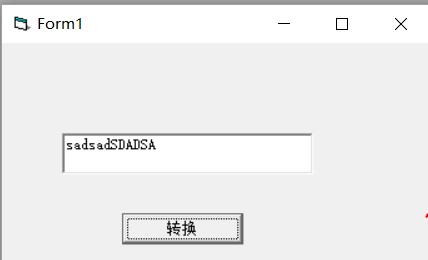答案：

Private Sub C1_Click()

For i = 1 To Len(Text1.Text)

a = Mid(Text1.Text, i, 1)              ‘一个一个选择text1中的字符

For j = 97 To 122

If a = Chr(j) Then                   ‘判断是不是小写字母

f = 0                             ‘是的话f=0

Exit For                          ‘并且要停止循环，不然会一直循环下去导致f=1

Else                             ‘否则f=1，也就是大写字母

f = 1

End If

Next

If f = 0 Then                        ‘如果是f=0（也就是字母为小写的情况）

a = UCase(a)                        ‘就转大写

End If                             ‘结束

If f = 1 Then                         ‘如果f=1（也就是大写的情况）

a = LCase(a)                         ‘就转小写

End If

b = b & a                           ‘全部a都赋值给b

Next

Text1.Text = b                         text1输出b

End Sub

三、三角形的综合问题。

根据给定的图形的三边的边长来判断图形的类型。若为三角形则同时计算出何种三角形，及三角形的周长和面积。要求完成”判断并计算”按钮的如下功能：

1、判断输入的条件是否为三角形，若是三角形则在Text1中显示“是三角形”；在Text2中显示是何种三角形。

2、单击“清除重来”按钮可以将所有的显示框清空，且按钮本身变为不可选取状态。当单击“判断并计算”之后重新恢复为可选状态。

提示：1.三角形存在的条件为任一边不为0且任两边之和大于第三边。

2.若一边具有a^2+b^2=c^2，则为直角三角形；若所有边具有a^2+b^2>c^2,则为锐角三角形；若一边具有a^2+b^2<c^2,则为钝角三角形。

3、三角形的面积公式.已知三角形三边a,b,c，则

（海伦公式）

（p=(a+b+c)/2）

S=sqrt[p(p-a)(p-b)(p-c)]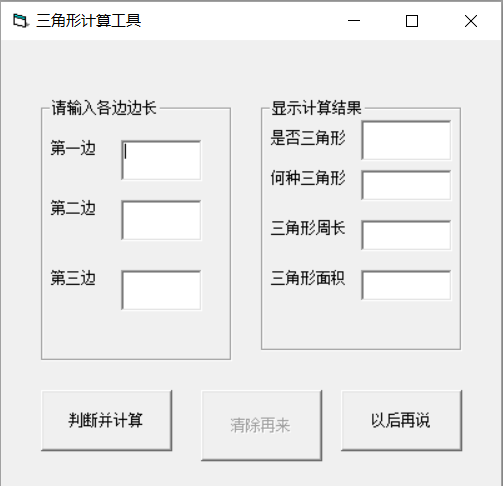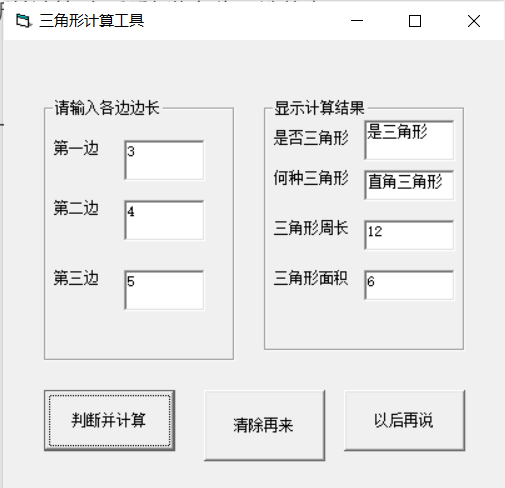答案：

Private Sub Command1_Click()

a = Val(Text1.Text)

b = Val(Text2.Text)

c = Val(Text3.Text)

Command2.Enabled = True

If a + b > c And a + c > b And c + b > a Then

Text4.Text = "是三角形"

Else

Text4.Text = "不是三角形"

End If

If Text4.Text = "是三角形" Then

If a ^ 2 + b ^ 2 = c ^ 2 Or a ^ 2 + c ^ 2 = b ^ 2 Or b ^ 2 + c ^ 2 = a ^ 2 Then

Text5.Text = "直角三角形"

End If

If a ^ 2 + b ^ 2 > c ^ 2 And a ^ 2 + c ^ 2 > b ^ 2 And b ^ 2 + c ^ 2 > a ^ 2 Then

Text5.Text = "锐角三角形"

End If

If a ^ 2 + b ^ 2 < c ^ 2 Or a ^ 2 + c ^ 2 < b ^ 2 Or b ^ 2 + c ^ 2 < a ^ 2 Then

Text5.Text = "钝角三角形"

End If

Text6.Text = a + b + c

Text7.Text = (1 / 4) * Sqr((a + b + c) * (a + b - c) * (a + c - b) * (b + c - a))

End If

End Sub

Private Sub Command2_Click()

Text1.Text = ""

Text2.Text = ""

Text3.Text = ""

Text4.Text = ""

Text5.Text = ""

Text6.Text = ""

Text7.Text = ""

End Sub

Private Sub Command3_Click()

End

End Sub

四、杨辉三角形。

编写适当的事件过程，在窗体上输出相对应行数的杨辉三角形，点击窗体弹出inputbox对话框，在对话框中输入行数，打印出如下图所示的杨辉三角形

会做这题，说明二维数组输出掌握很好。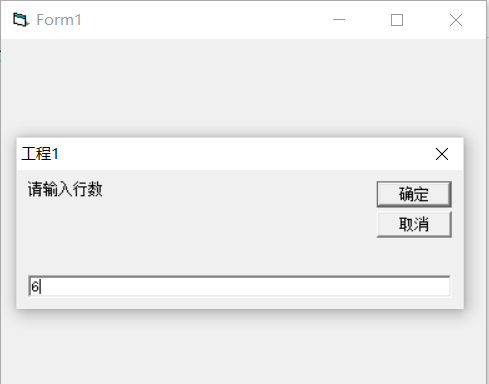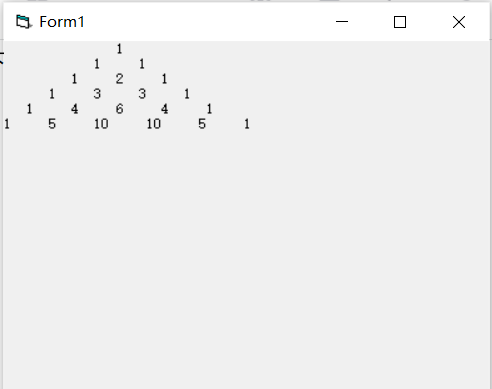答案：

Private Sub Form_Click()

Dim i As Integer, j As Integer

Dim a As Integer

a = Val(InputBox("请输入行数"))

Dim c(9, 9) As Integer

If a > 0 Then

For i = 1 To a

Print Space(3 * a - 3 * i);

For j = 1 To i

If i = 1 Or j = i Then       ‘当为三角形两边的时候，输出1

c(i, j) = 1

End If

If i = 2 Or j = i - 1 Then              ‘当为三角形的两边里面一个的时候输出行数-1

c(i, j) = i - 1

End If

If i > 2 And j < i - 1 Then                    否则都以自身上面一行对角线两个数的和。

c(i, j) = c(i - 1, j - 1) + c(i - 1, j)

End If

Print c(i, j) & "     ";                       ‘输出

Next

Print

Next

End If

End Sub

五、标签左右移动。

点击开始按钮，标签右移动，到达右边则向左移动，到达左边则向右移动，点击停止按钮则停止移动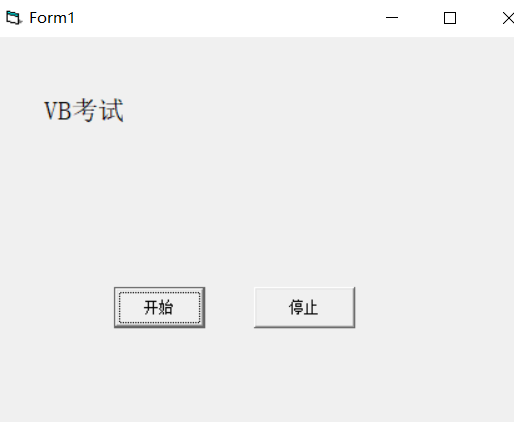答案：

Dim a

Private Sub Command1_Click()

Timer1.Enabled = True

End Sub

Private Sub Command2_Click()

Timer1.Enabled = False

End Sub

Timer1.Enabled = False

a = 0

End Sub

Private Sub Timer1_Timer()

If Label1.Left >= Val(Form1.Width) - Val(Label1.Width) Then

a = 1

End If

If Label1.Left <= 0 Then

a = 0

End If

If a = 1 Then

Label1.Left = Label1.Left - 100

End If

If a = 0 Then

Label1.Left = Label1.Left + 100

End If

End Sub

六、相邻数加起来为素数。

本程序用于求1~10中相邻数之和是素数的所有自然数对。

例如：1和2为相邻数并且1+2=3，3为素数，则输出1和2.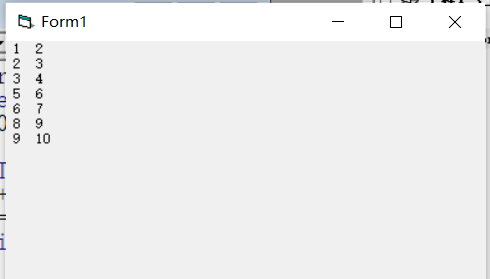答案：

Private Sub Form_Click()

Dim i As Integer

For i = 1 To 10

f = 0

For j = 2 To i - 1

If (i + (i + 1)) Mod j = 0 Then

f = 1

Exit For

Else

f = 0

End If

Next

If f = 0 Then

Print i; i + 1

End If

Next

End Sub

七、通项分母(累加).

下面程序的功能是计算，若通项分母的值>=200，停止计算（正确答案是S=1.409524

S=1+1/1*3 + 1/1*3*5 +1/1*3*5*7…..

答案：

Private Sub Form_Click()

Dim i As Integer

Dim n As Integer

i = 1

n = 1

Do

If n > 200 Then

Exit Do

Else

Print i

Sum = Sum + (1 / n)

End If

i = i + 2

n = n * i

Loop

Print Sum

End Sub

第二种：

Private Sub Form_Click()

Dim i As Integer

Dim n As Integer

i = 1

n = 1

Do While n <= 200

Sum = Sum + (1 / n)

i = i + 2

n = n * i

Loop

Print Sum

End Sub

八、判断降序数。

在文本框中输入一个整数数，判断是否为降序数。（降序数是高位的数比低位的数要大，依次降序，如3216549876等。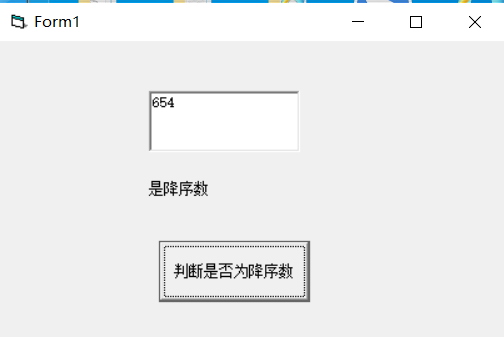答案：

Private Sub Command1_Click()

Dim a As String

a = Text1.Text

f = 0

If Val(a) > 0 And Val(a) = Int(Val(a)) Then

For i = 2 To Len(a)

If Val(Mid(a, i, 1)) <= Val(Mid(a, i - 1, 1)) Then   '当题目没给给定多少位，就是说不能使用数 组来做，则可以直接吧mid当做数组

f = 1

Else

f = 0

Exit For

End If

Next

If f = 1 Then

Label1.Caption = "是降序数"

Else

Label1.Caption = "不是降序数"

End If

Else

MsgBox "请输入正整数"

End If

End Sub

九、产生数组，求最大数。

点击窗体，用inputbox输出9个整数，放入数组a中并输出在窗体上，本程序按序号依次将（a1,a2,a3,(a2,a3,a4),…..(a9,a1,a2),九组元素分别相加（每组3个元素），生产新数组b并输出在窗体上，然后找出b数组中的最大元素并输出在窗体上。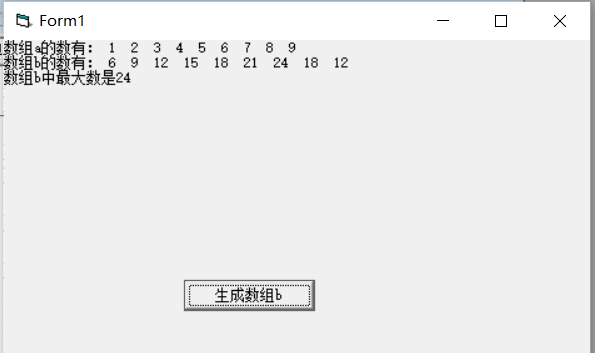答案：

Dim a(1 To 9) As Integer

Dim b(1 To 9) As Integer

Private Sub Command1_Click()

Dim c1, c2, c3

Print "数组b的数有：";

For i = 1 To 9

c1 = i

c2 = i + 1

c3 = i + 2

If c2 > 9 Then

c2 = c2 - 9

End If

If c3 > 9 Then

c3 = c3 - 9

End If

b(i) = a(c1) + a(c2) + a(c3)

Print b(i);

Next

Print

For i = 1 To 9

If b(i) > Max Then

Max = b(i)

End If

Next

Print "数组b中最大数是" & Max

End Sub

Private Sub Form_Click()

Print "数组a的数有：";

For i = 1 To 9

a(i) = Val(InputBox("请输入第" & i & "个数"))

Print a(i);

Next

Print

End Sub

十、奇偶排序。

随机生成9个数（范围为1~99）的数列，输出在窗体上，新数列的规则是：序列左边是奇数，序列右是偶数，奇、偶数分别从序列的两端开始依次向序列中间排放。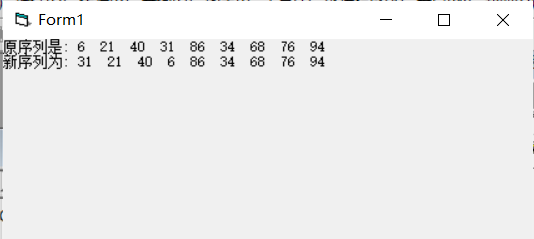答案：

Private Sub Form_Click()

Dim a(1 To 9)

Dim b(1 To 9)

Randomize

Print "原序列是:";

For i = 1 To 9

a(i) = Int(Rnd * (100 - 1 + 1) + 1)

Print a(i);

Next

Print

Print "新序列为:";

For i = 1 To 9

For j = 9 To i + 1 Step -1

If a(i) Mod 2 = 0 And a(j) Mod 2 <> 0 Then

t = a(i)

a(i) = a(j)

a(j) = t

End If

Next

Print a(i);

Next

End Sub

总结：

这是我发的第三份VB练习题，有同学能在不看答案的情况下能把三份的题全部做出来，说明你的VB这一块非常强了，在班级里应该是大神级的人物，考试对于你来说就是送分题。

当然有些基础不是很好，有些题是做不出来的同学也不要灰心，但是一定要去多自己尝试着做，别看一下题目就去看答案，这是没有用的。实在是想不出来的情况下在看看答案，理清答案的做题思路。千万不要去背答案哦，要理清答案思路，以后碰见了类似的题能自己做出来。

我在做这些题的时候是没有答案的，这些题的答案是我自己想出来的，我遇到了难题都是考自己想出来的，有些难题我花了一两个小时才写出来。

一开始我也是做题比较慢，经过不断的练习，做题速度就慢慢快了起来。

因为我发的题都是一些比较难的综合题，不会做也很正常，当然要是会做，那就更好了。

遇到一些不懂的地方可以私信或者评论哦！

展开全文weixin_53146084 2021-05-15 14:03:35
• 74KB jie123shao 2012-11-29 20:38:01...